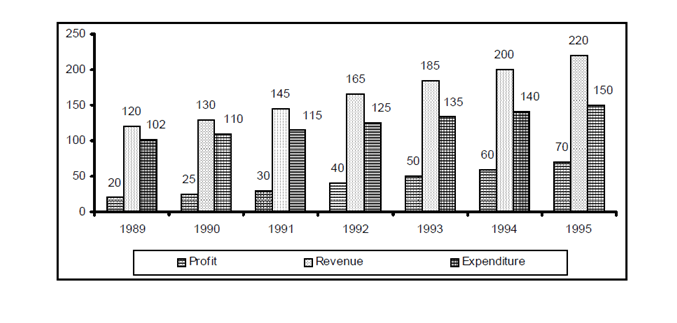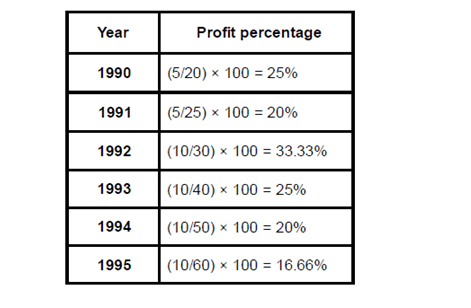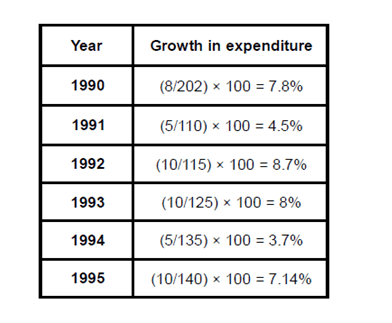Direction for questions 1 to 5: Answer the questions based on the following informationQuestion 1 : The average profit made in the given 7 years is approximately
a. Rs. 44 lakh
b. Rs. 42 lakh
c. Rs. 61 lakh
d. Rs. 56 lakh

Question 2 : The expenditure for the 7 years together form what per cent of the revenues during the same period?
a. 75%
b. 67%
c. 62%
d. 83%

Question 3 : Which year showed the lowest percentage increase in profit as compared to the previous year?
a. 1993
b. 1994
c. 1990
d. 1995

Question 4 : In which year was the growth in expenditure maximum as compared to the previous year?
a. 1993
b. 1995
c. 1991
d. 1992

Question 5 :  If the profit in 1996 shows the annual rate of growth as it had shown in 1995 over the previous year, then what approximately will be the profit in 1996?
a. Rs. 72 lakh
b. Rs. 82 lakh
c. Rs. 93 lakh
d. Rs. 78 lakh

Average profit over the period of 7 years = (20 +25 +30+ 40 +50 +60 +70)/7 = 42.14

Approximately is Rs. 42 lakh.

Expenses of 7 years = (102 + 110 + 115 + 125 + 135 + 140 + 150) = 877

Revenue of 7 years = (120 + 130 + 145 + 165 + 185 + 200 + 220) = 1165.

∴ required percentage = 877/1165× 100 = 75%approx.

Profit percentage = (Present profit – previous profit) / previous profit × 100

The table below shows the profit percentage for each yearThe lowest percentage increase in profit as compared to the previous year can be observed in the year 1995 which is 16.66%.

Growth in expenditure over the previous year = (previous expenditure – present expenditure) / previous expenditure × 100The growth in expenditure is maximum as compared to the previous year in the year 1992 i.e. 8.7%.